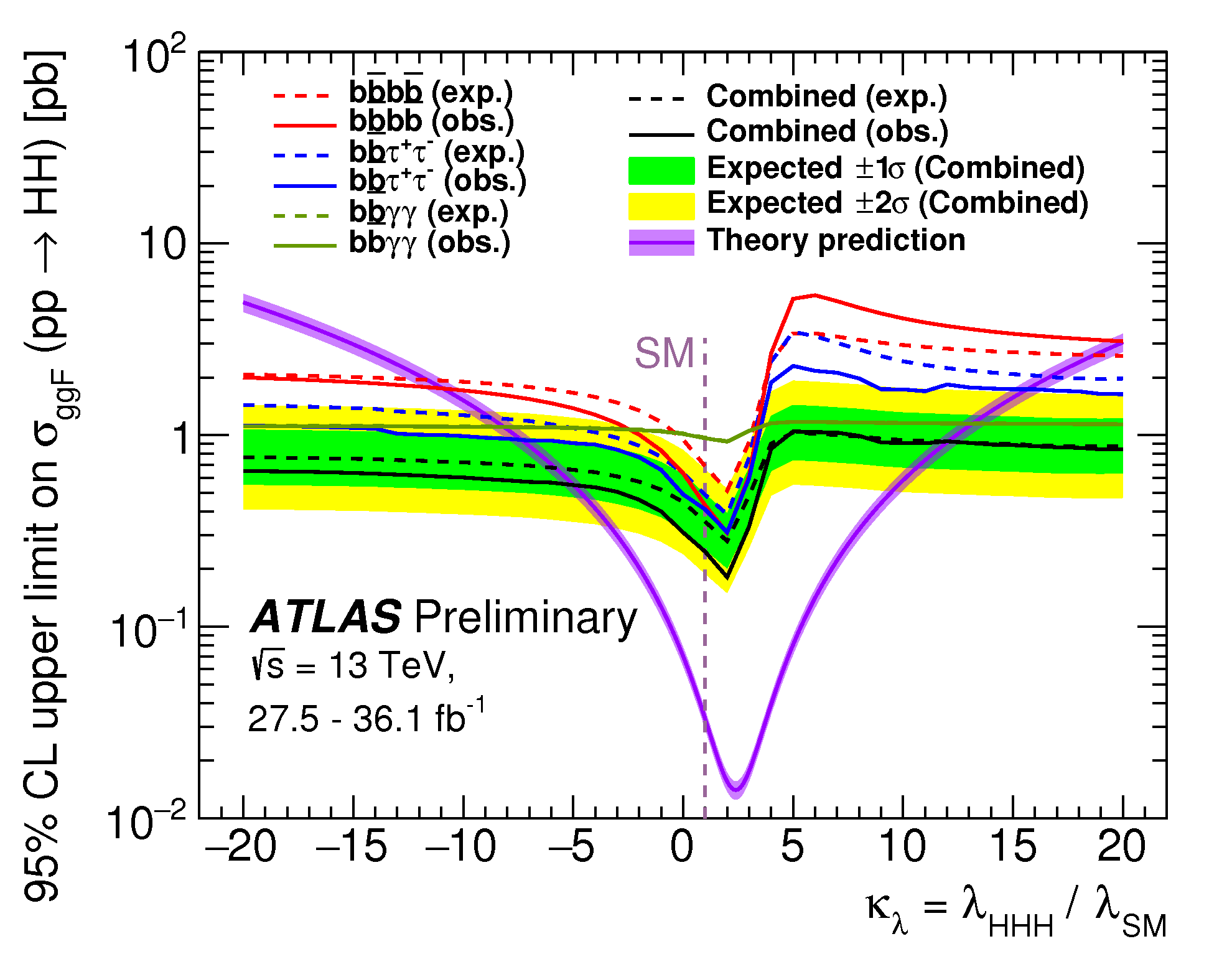Upper limits at 95% confidence level on the cross-section of the non-resonant Higgs boson pair production as a function of κλ. The allowed range of κλ is derived from the interval where the theoretical prediction is found below the experimental upper limits on the cross-section. (Image: ATLAS Collaboration/CERN)

The Brout-Englert-Higgs (BEH) mechanism is at the core of the Standard Model, the theory that describes the fundamental constituents of matter and their interactions. It introduces a new field, the Higgs field, through which the weak bosons (W+/- and Z) become massive while the photon remains massless. The excitation of this field is a physical particle, the Higgs boson, which was discovered by the ATLAS and CMS collaborations in 2012.

The BEH mechanism also predicts that the Higgs field can interact with itself; in other words, a single (virtual) Higgs boson can decay into two Higgs bosons. Observing and measuring this self-interaction, or “Higgs self-coupling”, would be the ultimate validation of the theory of mass generation, while any deviation from Standard Model predictions would open a window on new physics.

Unfortunately, Higgs boson pairs are predicted to be very rare in proton–proton collisions, with a production rate roughly a thousand times smaller than the single Higgs boson. To make matters worse, not all di-Higgs boson production occurs through Higgs self-coupling. Vast amounts of data are therefore needed for this to be probed, making it a flagship analysis for the high-luminosity upgrade of the LHC (HL-LHC). It is nevertheless important to explore di-Higgs production also with smaller datasets as new physics beyond the Standard Model might enhance the production rate.

### The new ATLAS result provides the world’s most stringent constraint on the anomalous Higgs self-coupling to date.

The ATLAS collaboration has searched for Higgs boson pairs (HH) in the dataset collected in 2015 and 2016 using various decay channels. The most sensitive of these involve one Higgs boson decaying into a pair of b-quarks and one decaying into either another pair of b-quarks (HH→bbbb), two tau-leptons (HH→bbττ) or two photons (HH→bbγγ). These three searches were recently statistically combined and, as a result, the production rate of HH pairs could be excluded beyond 6.7 times the Standard Model prediction, at a 95% confidence level.

New physics could be indicated by a Higgs self-coupling which differs from the Standard Model prediction by a factor κλ. This would affect the production rate and the kinematic distributions of the Higgs boson pairs and, as such, is an excellent probe for new physics. The recent statistical combination of the HH searches in ATLAS constrains the value of κλ to be between –5.0 and +12.1, at a 95% confidence level (see figure). It is the world’s most stringent constraint on the anomalous Higgs self-coupling to date.

Higgs boson pairs are also a key signature of heavy new particle decays in several scenarios beyond the Standard Model. These might include an additional Higgs boson in models that extend the Higgs sector of the Standard Model, or the excitation of a graviton in models with extra spatial dimensions. The combined HH searches performed by ATLAS with the 2015-2016 dataset impose stringent constraints on the production rates of such resonances at the LHC.